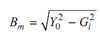## Open Circuit Test Assignment Help

Assignment Help: >> Open Circuit Test and Short Circuit Test - Open Circuit Test

Open Circuit Test:

This test is performed to determine the core losses and shunt branch parameters Gi and Bm  of the equivalent circuit of the transformer. This test is performed on the low voltage winding side whereas high voltage winding is kept open circuited. The connection diagram is illustrated in the Figure. Rated voltage is applied to the low voltage winding and meter readings are recorded.

Amps Power input = P0 Watts

The no load current I0 drawn by the transformer in very low usually 2-6% of the rated current. The winding resistances and reactances are of extremely low ohmic value. Thus, ohmic losses may be neglected.

The equivalent (approximate) circuit is illustrated in FigureFigure: Equivalent Circuit for Open Circuit

The watt meter reads the core losses (P0), watts :

(V1 )2 Gi  = P0

Gi  =     P0 /(V1 )2

Y  = I0 /V1We must know the Gi and Bm are referred to the low voltage winding side. We may easily transform them to the HV winding side.